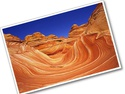## Surface Area and Volume

Type of Unit: Conceptual

# Prior Knowledge

Students should be able to:

• Identify rectangles, parallelograms, trapezoids, and triangles and their bases and heights.
• Identify cubes, rectangular prisms, and pyramids and their faces, edges, and vertices.
• Understand that area of a 2-D figure is a measure of the figure's surface and that it is measured in square units.
• Understand volume of a 3-D figure is a measure of the space the figure occupies and is measured in cubic units.

# Lesson Flow

The unit begins with an exploratory lesson about the volumes of containers. Then in Lessons 2–5, students investigate areas of 2-D figures. To find the area of a parallelogram, students consider how it can be rearranged to form a rectangle. To find the area of a trapezoid, students think about how two copies of the trapezoid can be put together to form a parallelogram. To find the area of a triangle, students consider how two copies of the triangle can be put together to form a parallelogram. By sketching and analyzing several parallelograms, trapezoids, and triangles, students develop area formulas for these figures. Students then find areas of composite figures by decomposing them into familiar figures. In the last lesson on area, students estimate the area of an irregular figure by overlaying it with a grid. In Lesson 6, the focus shifts to 3-D figures. Students build rectangular prisms from unit cubes and develop a formula for finding the volume of any rectangular prism. In Lesson 7, students analyze and create nets for prisms. In Lesson 8, students compare a cube to a square pyramid with the same base and height as the cube. They consider the number of faces, edges, and vertices, as well as the surface area and volume. In Lesson 9, students use their knowledge of volume, area, and linear measurements to solve a packing problem.

Subject:
Mathematics, Geometry
Material Type:
Unit of Study
Level:
Middle School
6
Tags:
Cubes, Surface Area, Trapezoids, Volume, Vertices, Square, 6th Grade Mathematics, Faces, Formulas, Cube, Grids, Rectangular Prisms, Pyramid, Composite Figures, Prisms, Edges, Parallelograms, Measurement, Problem-Solving, Triangles, 3d

Lesson 1

· 8 1/2 x 11 in. paper (two sheets for each pair of students)

· Tape (one for each pair of students)

Lesson 4

· Composite Figure handout (one for each pair of students)

· Rulers and pencils (one for each pair of students)

· Scissors, optional (one for each pair of students)

Lesson 7

• Grid paper and scissors
• Cubes (optional)

Lesson 8

· Inch grid paper (one sheet for each pair of students)

· Scissors (one for each pair of students)

Unit 9 Surface Area and Volume•••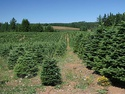•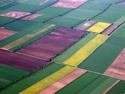•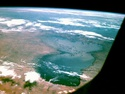•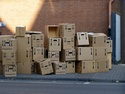•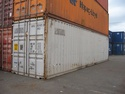•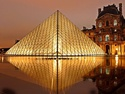•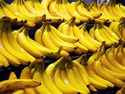•